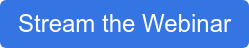Engineers often need to answer questions regarding heat transfer in their designs. As with many other things, the specific task or question being asked will determine what tool to use to get the best result. Here we look at answering the question of when to use Finite Element Analysis (FEA) vs. Computational Fluid Dynamics (CFD) in heat transfer problems.

#### Heat Transfer

Heat can be transferred by 3 different methods: conduction (solids), convection (fluid or gas) and radiation.Understanding your particular problem and knowing what capabilities FEA or CFD software provide are key to knowing which path to take.

#### Thermal Analysis with FEA Software

A thermal analysis performed with SOLIDWORKS Simulation (FEA) will allow you to input convection coefficient values, either as a constant or as a constant variable with respect to time or temperature. You may also define solid interface thermal resistance or input radiation effects based on ambient temperature, surface emissivity and view factor.

What this means is that when it comes to thermal analyses and FEA, you need to be certain that your convective effects are predictable and can be accurately described by the above information. Predictable effects can be defined as conditions where you have forced convection and honest convective coefficient values.

#### Convection

Convection can be described by a global convective coefficient (FEA) or by a local differential method on the fluid boundary side where the normal gradient of the fluid-temperature field and fluid conductivity are analyzed (CFD). When flow effects (in fluid or gas) are unpredictable and you are uncertain of boundary conditions or coefficients of convection, or when calculating the effects of natural convection, turn to CFD.

With respect to radiation and FEA, all surfaces in the analysis must be defined with accurate emissivity, view factors and ambient conditions. This can many times translate into a rather involved process that could lead to a reduction in your workflow efficiency. CFD is more robust (especially combined with the SOLIDWORKS Flow Simulation HVAC module) when radiation effects are not negligible, as it can account for more radiation factors, such as spectral dependencies, absorption in semi-transparent solids, smooth distribution of the radiative heat fluxes, solar radiation, etc.SOLIDWORKS Simulation (FEA) SOLIDWORKS Flow Simulation (CFD)

#### Thermal Analysis with CFD

SOLIDWORKS Flow Simulation (CFD) makes it incredibly easy to input all the necessary data to make a prediction for all three modes of heat transfer. Speaking again from a practical standpoint, if you have a rather complex model and plan to input convective coefficients, conductive resistances and known surface-to-surface or surface-to-ambient radiation effects in an FEA analysis, CFD will be much quicker to set up. On the other hand, if you have predictable, forced convection and negligible radiation effects, FEA will run the problem faster.

With all that being said, let’s make this question a bit easier to answer:

Does your analysis involve known convection coefficients or is it a conduction only problem? Is radiation negligible? Are you unconcerned about what is happening in the fluid region? Is the primary convective mode forced convection instead of natural convection? If you answered yes to all these questions, then use FEA. For every other situation, use CFD.

Interested in learning more SOLIDWORKS Simulation? Watch our on-demand webinar, Considerations When Designing for Simulation!